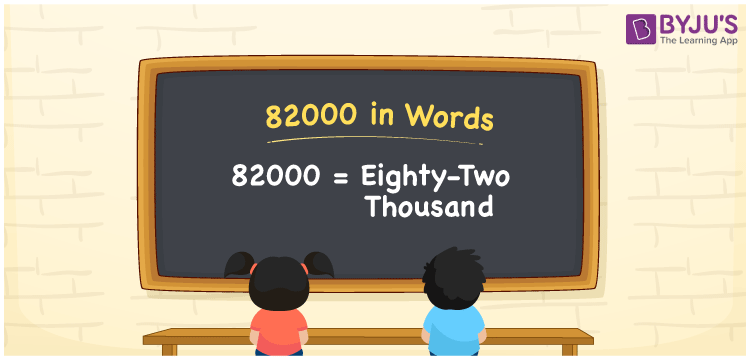# 82000 in Words

The number 82000 stands for Eighty-two Thousand. The place value chart is useful when converting numbers to words. As a result, you can say that when writing 82000 in words, employing the place value table is advantageous. For instance, if you gained Rs. 82000 in 8 months, you may write “I gained Rs. Eighty-two Thousand in eight months”. Let’s have a look at how to spell 82000 in words and the procedures that are required, along with a detailed explanation.

 82000 in Words: Eighty-two Thousand. Eighty-two Thousand in Numerical Form: 82000.

## 82000 in English Words## How to Write 82000 in Words?

A place value chart can be used to convert 82000 to words. A place value table for the number 82000, as well as an expanded version, is provided below.

 Ten-thousands Thousands Hundreds Tens Ones 8 2 0 0 0

The number 82000 is represented as follows using a place value chart:

= 8 × Ten thousand + 2 × Thousand + 0 × Hundred + 0 × Ten + 0 × One

= 8 × 10000 + 2 × 1000

= 80000 + 2000

= 82000

= eighty-two thousand

Hence, 82000 in words is eighty-two thousand.

The natural number 82000 occurs after 81999 but before 82001.

82000 in words – Eighty-two Thousand

Is 82000 an odd number? – No

Is 82000 an even number? – Yes

Is 82000 a perfect square number? – No

Is 82000 a perfect cube number? – No

Is 82000 a prime number? – No

Is 82000 a composite number? – Yes

## Frequently Asked Questions on 82000 in Words

Q1

### How to write 82000 in words?

82000 in words is eighty-two thousand.

Q2

### Simplify 83500 – 1500, and express in words.

Simplifying 83500 – 1500, we get 82000. Hence, 82000 in words is eighty-two thousand.

Q3

### How to write eighty-two thousand in numbers?

eighty-two thousand in numbers is 82000.